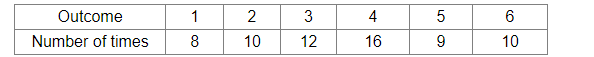# The outcomes of 65 throws of a dice were noted as shown below

Question:

The outcomes of 65 throws of a dice were noted as shown belowA dice is thrown at random. What is the probability of getting a prime number?

(a) $\frac{3}{35}$

(b) $\frac{3}{5}$

(c) $\frac{31}{65}$

(d) $\frac{36}{65}$

Solution:

(c) $\frac{31}{65}$

Explanation:
Total number of throws = 65
Let E be the event of getting a prime number.
Then, E contains 2, 3 and 5, i.e. three numbers.

$\therefore P($ getting a prime number $)=P(E)=\frac{\text { Number of times prime number s occur }}{\text { Total number of throws }}=\frac{(10+12+9)}{65}=\frac{31}{65}$